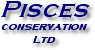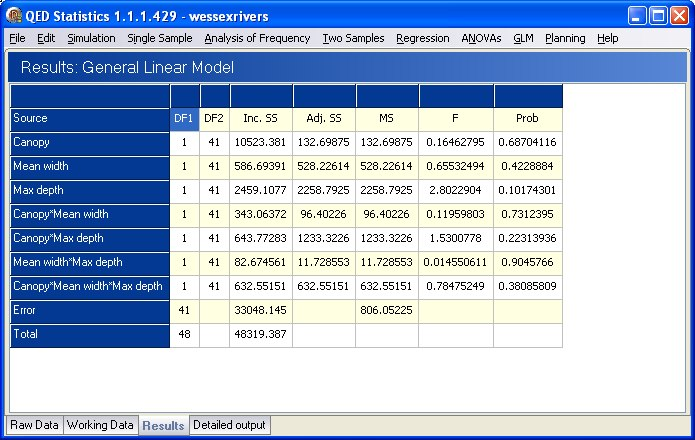General Linear Models: The modern approach that combines ANOVAs and regression

The GLM model builder lets you define as simple or complex a model as you need:

• Combines ANOVA, ANCOVA, MANOVA, MANCOVA, ordinary linear regression, t-Test and F Test.
• 1 dependent and unlimited independent variables.
• Multiple interactions between independent variables.
• Mix categorical and continuous variables.
• 3 coding schemes for categorical variables.Intuitive and easy-to-use setup procedure guides you through the building of the most complex GLM

Detailed output shows the full calculations for each variable and step of the model